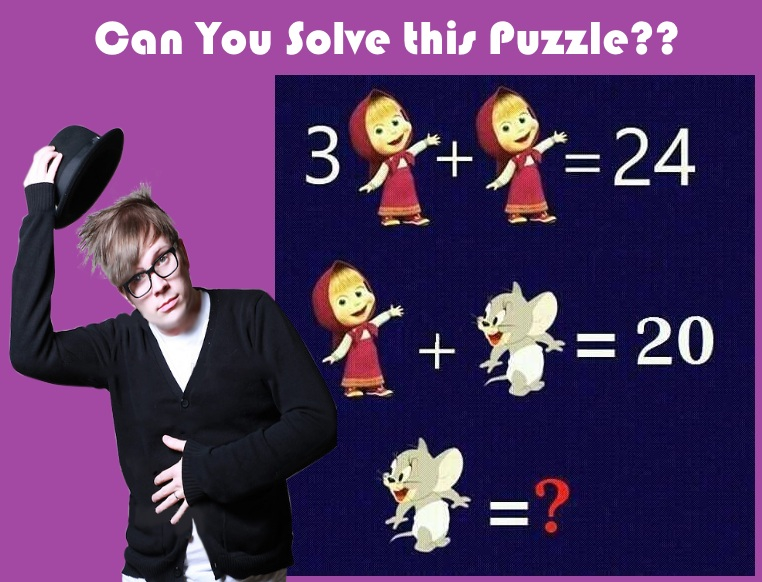This post may contain affiliate links. This means if you click on the link and purchase the item, We will receive an affiliate commission at no extra cost to you. See Our Affiliate Policy for more info.

# Find Out the Value of MOUSE?? – COOL Math Brainteaser with Answers

0
1462

Hello Math Geniuses, Here’s an interesting math puzzle you to solve. You think you are smart enough? lets take a math test.

In this Cool brainteaser, Two equation is given made by DOLL and MOUSE. You have to find out the Value of MOUSE?

## DOLL and MOUSE COOL Math Puzzle – Only For Math Geniuses

If you failed to solve this puzzle. Don’t worry answer and solution given below.

# Find Out the Value of MOUSE??Solve it? Found the Answer?? See you in the comment…,

Let’s see You are Right or Not??

First, Let’s Consider   ” DOLL = a “  and  ” MOUSE = b “

Equation(1)

3 a + a = 24

4 a = 24

a = 6

DOLL = 6

Equation(2)

a + b = 20

6 + b = 20

b = 14

MOUSE = 14